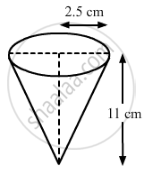# Sushant has a vessel, of the form of an inverted cone, open at the top, of height 11 cm and radius of top as 2.5 cm and is full of water. Metallic spherical balls each of diameter 0.5 - Mathematics

Sushant has a vessel, of the form of an inverted cone, open at the top, of height 11 cm and radius of top as 2.5 cm and is full of water. Metallic spherical balls each of diameter 0.5 cm are put in the vessel due to which 2/th of the water in the vessel flows out. Find how many balls were put in the vessel. Sushant made the arrangement so that the water that flows out irrigates the flower beds. What value has been shown by Sushant?

#### SolutionHeight (h) of the conical vessel = 11 cm
Radius (r1) of the conical vessel = 2.5 cm
Radius (r2) of the metallic spherical balls =0.5/2 =0.25 cm
Let n be the number of spherical balls that were dropped in the vessel.
Volume of the water spilled = Volume of the spherical balls dropped

2/5xx"Volume of cone" = nxx"Volume of one spherical ball"

⇒2/5xx1/3πr_1^2h = nxx4/3πr_2^3

⇒r_1^2h=nxx10r_2^3

⇒(2.5)^2×11=n×10×(0.25)^3

⇒68.75=0.15625n

⇒n=440

Hence, the number of spherical balls that were dropped in the vessel is 440.
Sushant made the arrangement so that the water that flows out, irrigates the flower beds. This shows the judicious usage of water.

Concept: Concept of Surface Area, Volume, and Capacity
Is there an error in this question or solution?
Chapter 14: Surface Areas and Volumes - Exercise 14.1 [Page 30]

#### APPEARS IN

RD Sharma Class 10 Maths
Chapter 14 Surface Areas and Volumes
Exercise 14.1 | Q 47 | Page 30
Share